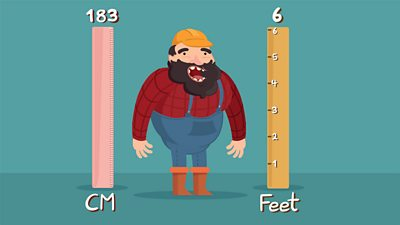# What are imperial measurements?

Miles, feet and inches are old units of length. These are known as imperial units of length but are not now commonly used in maths.

• There are 12 inches in a foot.
• An inch is roughly equal to 2.5 centimetres.
• A foot is roughly equal to 30 centimetres.
• A mile is roughly equal to 1.5 kilometres.

## There's more to learn...

This guide